Click to Chat

1800-1023-196

+91-120-4616500

CART 0

• 0

MY CART (5)

Use Coupon: CART20 and get 20% off on all online Study Material

ITEM
DETAILS
MRP
DISCOUNT
FINAL PRICE
Total Price: Rs.

There are no items in this cart.
Continue Shopping• Complete Physics Course - Class 11
• OFFERED PRICE: Rs. 2,968
• View Details

```Newton’s Third Law of Motion

Table of Content

Simulation for Newton's Third Law

Few Examples on Newton’s third Law of Motion

Related Resources

It states that,

“To every action there is an equal and opposite reaction”.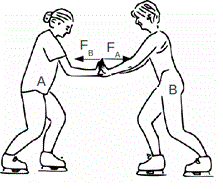Whenever one force acts on a body, it gives rise to another force called reaction. A single isolated force is an impossibility. The two forces involved in any interaction between two bodies are called “action” and “reaction”. But this does not imply any difference in their nature, or that one force is the ‘cause‘ and the other is the ‘effect’. Either force may be considered as ‘action’ and the other ‘reaction’ to it.

It may be noted that action and reaction never act on same body.

Note: The most important fact to notice here is that these oppositely directed equal action and reaction can never balance or cancel each other because they always act, on two different point (broadly on two different objects) For balancing any two forces the first requirement is that they should act one and the same object. (or point, if object can be treated as a point mass, which is a common practice)

Simulation for Newton's Third Law

Few Examples on Newton’s third Law of Motion

(a)  Book Kept on a Table

A book lying on a table exerts a force on the table which is equal to the weight of the book. This is the force of action. The table supports the book, by exerting an equal force on the book. This is the force of reaction, as shown in the below figure. As the system is at rest, net force on it is zero. Therefore, forces of action and reaction must be equal and opposite.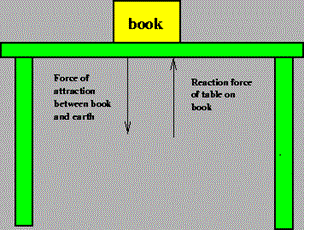(b) Walking on the ground:-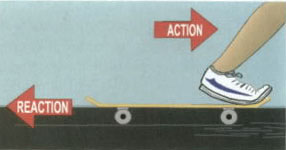While walking a person presses the ground in the backward direction (action) by his feet. The ground pushes the person in forward direction with an equal force (reaction). The component of reaction in the horizontal direction makes the person move forward.

(c) Process of Swimming:-

A swimmer pushes the water backwards (action). The water pushed the swimmer forward (reaction) with the same force. Hence the swimmer swims.

(d) Firing from a gun:-

When a gun is fired, the bullet moves forward (action). The gun recoils backwards (reaction).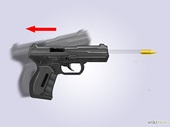(e) Fight of jet planes and rockets:-

The burnt fuel which appears in the form of hot and highly compressed gases escapes through the nozzle (action) in the backward direction. The escaping gases push the jet plane or rocket forward (reaction) with the same force, hence, the jet or rocket moves.

(f) Rubber ball re-bounds from a wall:-

When a rubber ball is struck against a wall or floor it exerts a force on a wall (action). The ball rebounds with an equal force (reaction) exerted by the wall or floor on the ball.

(g) It is difficult to walk on sand or ice:-

This is because on pushing, sand gets displaced and reaction from sandy ground is very little. In case of ice, force of reaction is again small because friction between feet and ice is very small.

(h) Driving a nail in to a wooden block without holding the block is difficult:-

This is because when the wooden block is not resting against a support, the block and nails both move forward on being hit with a hammer. However, when the block is held firmly against a support, and the nail is hit, an equal reaction of the support drives the nail into the block.

(i) A tea cup breaks on falling on the ground:-

Tea cup exerts certain force (action) on ground while the ground exerts an equal and opposite reaction on the cup. Ground is able to withstand the action of cup, but the cup being relatively more delicate breaks due to reaction.

Problem 1:-

Two blocks, with masses m1 = 4.6 kg and m2 = 3.8 kg, are connected by a light spring on a horizontal frictionless table. At a certain instant, when m2 has an acceleration a2 = 2.6 m/s2, (a) what is the force on m2 and (b) what is the acceleration of m1?

Concept:-

Force acting on the body (F) is equal to the product of mas of the body (m) and acceleration of the body (a).

So, F = ma

From equation F = ma, the acceleration (a) of the body would be,

a = F/m

Solution:-

(a) The net force ∑ Fx on the second box having mass m2 will be,

∑ Fx = m2a2x

Here a2x is the acceleration of the second block.

To obtain the net force ∑ Fx on the second box having mass m2, substitute 3.8 kg mass m2 and 2.6 m/s2 for a2x in the equation ∑ Fx = m2a2x,

∑ Fx = m2a2x

= (3.8 kg) (2.6 m/s2)= 9.9 kg .m/s2

= (9.9 kg .m/s2) (1 N/ 1 kg .m/s2)= 9.9 N

From the above observation we conclude that, the net force ∑ Fx on the second box having mass m2 would be 9.9 N. There is only one (relevant) force on the block, the force of block 1 on block 2.

(b) There is only one (relevant) force on block 1, the force of block 2 on block 1. By Newton’s third law this force has a magnitude of 9.9 N.

So the Newton’s second law gives,

∑ Fx = m1a1x = -9.9 N

But, m1a1x = (4.6 kg) (a1x)       (Since, m1 = 4.6 kg)

(4.6 kg) (a1x) = -9.9 N

So, a1x = -9.9 N/4.6 kg

= (- 2.2 N/kg) (1 kg.m/s2 / 1 N) = -2.2 m/s2

From the above observation we conclude that, the acceleration of m1 will be -2.2 m/s2.

_______________________________________________________________________________________________

Problem 2:-

A meteor of mass 0.25 kg is falling vertically through Earth’s atmosphere with an acceleration of 9.2 m/s2. In addition to gravity, a vertical retarding force (due to frictional drag of the atmosphere) acts on the meteor as shown in the below figure. What is the magnitude of this retarding force?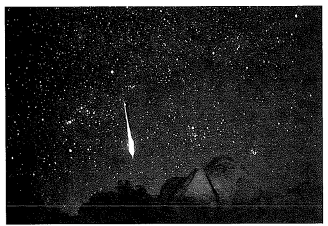Solution:-

Given Data:

Mass of the meteor, m = 0.25 kg

Acceleration of the meteor, a = 9.2 m/s2

The net force exerted (Fnet) on the meteor will be,

Fnet = ma

= (0.25 kg) (9.2 m/s2) = (2.30 kg. m/s2) (1 N/ 1 kg. m/s2) = 2.30 N      …… (1)

If g (g = 9.80 m/s2) is the free fall acceleration of meteor, then the weight of the meteor (W) will be,

W = mg = (0.25 kg) (9.80 m/s2)

= (2.45 kg. m/s2) (1 N/ 1 kg. m/s2) = 2.45 N        …… (2)

The vertical retarding force would be equal to the net force exerted on the meteor (Fnet) minus weight of the meteor (W).

So, vertical retarding force = Fnet –W          …… (3)

Putting the value of Fnet and W in equation (3), the vertical retarding force will be,

Vertical retarding force = Fnet –W = 2.30 N -2.45 N = -0.15 N        ……. (4)

From equation (4) we observed that, magnitude of the vertical retarding force would be, -0.15 N.

_______________________________________________________________________________________________

Problem 3:-

Suppose in figure shown above we put one more block of 5 kg mass adjacent to 10 kg and a force of 150 N acts as shown in the figure below, then find the forces acting on the interface.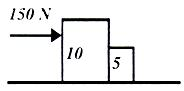Solution:-

The combined acceleration of the two bodies when treated as one is
a = F/((10+5))=150/15=10/sec2

So each one moves with a = 10m/sec2 keeping their contact established.

Here you can feel that due to 150N force the body of 5 kg feels as if it is being pushed by the 10 kg mass. There is force acting on 5kg called R1, to oppose it by third law this body exerts a force R2 on 10kg. The interface is as shown in Figure given below.Also, third law tells us that R1 = R2 in magnitude and is opposite in direction.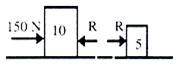R1 = R2 = R

Here since 150 N force acts on the 10kg mass and only r acts on the 5kg mass. For motion in 5kg only R is responsible. We can write the initial equation as:

F = 150 = (10 + 5) a

150 = 10a + 5a

Here 10a is force experienced by 10kg mass. And 5a is experienced by 5kg mass.

R = 5a   a = 10m/sec2

So,R = 50N

Thus,Net force experienced by 10kg block is (150-R) = 10a 150-R = 1010 = 100 N

Therefore, R = 50

Therefore we get R = 50N for both blocks. Hence we find "action and reaction are equal and opposite". Now net force on the body of 10kg mass is 100N & Net force on the body of 5kg mass is 50N and on the interface action and reaction are both equal and also are equal to force experienced by second body.

_________________________________________________________________________________________________

Problem 4:-

An object is hung from a spring scale attached to the ceiling of an elevator. The scale reads 65 N when the elevator is standing still. (a) What is the reading when the elevator is moving upward with constant speed of 7.6 m/s? (b) What is the reading of the scale when the elevator is moving upward with a speed of 7.6 m/s and decelerating at 2.4 m/s2?

Solution:-

Weight of the object (W) when the elevator is standing, W = 65 N

(a) We have to find out the scale reading when the elevator is moving upward with a constant speed of 7.6 m/s.

Since the elevator is moving upward with a constant speed, therefore there is no acceleration of the system resulting there is no force. Thus the scale reading must be equal to the weight of the object and that will be 65 N.

(b) We have to find out the scale reading when the elevator is moving upward with a speed of 7.6 m/s and decelerating at 2.4 m/s2.

The force exerted on the object due to the deceleration at the rate 2.4 m/s2 (a = - 2.4 m/s2) will be,

F = ma

= (W/g) a    …… (1)

Where W is the weight of the object (W = 65 N) when the elevator is at rest and g is the free fall acceleration of the object (g=9.80 m/s2).

Putting the value of W, g and a in equation (1) the force exerted on the object will be,

F = (W/g) a

= (65 N/9.80 m/s2) (-2.4 m/s2)

= -15.92 N

= -16 N                …… (2) (Rounding off to two significant figure)

When the elevator is moving upward with a speed of 7.6 m/s and decelerating at 2.4 m/s2, the force would be,

= F- (-W)

= -16 N –(-65 N)

= -16 N+65 N

= 49 N      …… (3)

From the above observation we conclude that, the scale reading when the elevator is moving upward with a speed of 7.6 m/s and decelerating at 2.4 m/s2 would be 49 N.

Related Resources

Look into the Sample Papers of Previous Years to get a hint of the kinds of questions asked in the exam.

You can get the knowledge of Useful Books of Physics.

To read more, Buy study material of Laws of Motion comprising study notes, revision notes, video lectures, previous year solved questions etc. Also browse for more study materials on Physics here.
```### Course Features

• 101 Video Lectures
• Revision Notes
• Previous Year Papers
• Mind Map
• Study Planner
• NCERT Solutions
• Discussion Forum
• Test paper with Video Solution